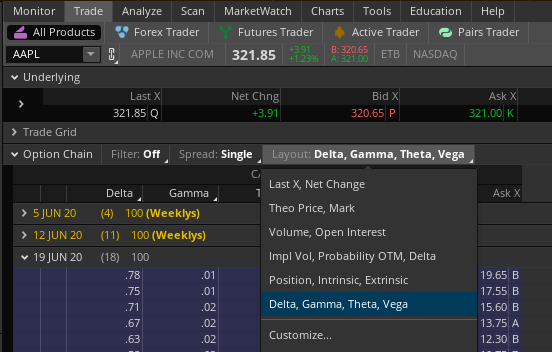top of page
•Admin

# Option Greeks Explained

If you are new to trading options, it all may seem a bit overwhelming, especially when you begin learning about the greeks. The five greeks (delta, gamma, vega, theta, and rho) are sensitivity measures that describe how the prices of options change. They are important because they describe the inputs into the Black-Scholes option pricing model, meaning that they each factor into the price of an option. Hopefully after reading this, you will have a better understanding of the greeks.How to View the Greeks in Thinkorswim

In order to see the greeks in Thinkorswim, click on the "Trade" tab to view the option chain. Next, click the "Layout" drop down menu and select the option for "Delta, Gamma, Theta, Vega." If you would also like to see Rho, you can click the "Customize" option and search for "Rho" to add it.

Below is an option chain for "SPY". When viewing the option chain, calls are on the left side, and puts are on the right side. The options highlighted in blue are in-the-money, and the ones with a black background are out-of-the-money. In my explanations below, I will be using the at-the-money C320 option as an example, which is the call with a strike price of \$320.Thinkorswim Option Chain

Delta

Delta is the most important of the greeks. It measures the sensitivity of an option price to the price of the underlying security. In other words, it is the amount by which the price of an option will change if the price of the underlying security changes \$1. In the option chain above, the C320 option has a Delta value of .47, meaning that if the price of SPY increases by \$1, the price of this option will increase by 47 cents. You will notice that Delta is higher the farther in-the-money an option is, and is smaller for out-of-the-money options. Also notice that Delta is negative for puts, which means the price of puts would decrease if the price of the underlying security increased.

Gamma

Of all five greeks, gamma is probably the most difficult to understand. If Delta is the derivative of the underlying security price, then Gamma is the second derivative. Gamma describes the change in Delta for a \$1 change in the price of the underlying security. For example, a Gamma value of .03 in the option chain above means that if the price of SPY increases by \$1, the value of Delta will increase by .03, resulting in a Delta value of .50 for the C320 option. Gamma is highest for the at-the-money-option, and decreases the farther in-the-money or out-of-the-money you go.

Vega

Vega is the sensitivity of an option to the volatility of the underlying security. A Vega value of .26 in the option chain above means that if volatility increases by 1%, the price of the option will go up by 26 cents. Notice that Vega is more or less the same for a call and a put with the same strike price. Calls and puts both become more expensive as volatility increases, and cheaper as volatility decreases.

Vega is highest for at-the-money options, and decreases the farther in-the-money or out-of-the-money an option is. Also, the longer the time to expiration of an option, the higher Vega will be. To explain why this is the case, suppose an option that expires in a week is deeply out-of-the-money. Since it is so far out-of-the-money, and there isn't much time for it to move to become in-the-money, this option will likely expire worthless, no matter how volatile the stock is. Vega will have a low value in this case. Now suppose an option is at-the-money and expires in six months. In this case, any movement in the stock could push the option into-the-money or out-of-the-money, and there is a lot of time for the stock to move. Because of this, this option will be much more sensitive to the stock's volatility, so Vega will be higher.

Theta

Theta is the time decay of an option. It measures the sensitivity of option prices to the amount of time remaining until the expiration date. For example, a Theta value of -.15 means that the option will be worth 15 cents less tomorrow than it was worth today, assuming all other factors stayed constant. Theta is negative for calls and puts, because they both lose value as time passes. Theta will be the highest for at-the-money options, just like Gamma and Vega.

Rho

Rho is the sensitivity of option prices to changes in interest rates. For example, a Rho value of .06 means that the price of a call will go up six cents if interest rates go up by one percent. Notice in the option chain above that Rho is positive for calls, and negative for puts; an increase in interest rates increases the value of calls, but decreases the value of puts. Both types of options are more sensitive to interest rates if they are in-the-money, and less sensitive if they are out-of-the-money. In addition, both types of options are more sensitive to interest rates if there is more time to expiration. Since interest rates change relatively slowly, Rho is the least important of the greeks.

This was a very basic and high-level summary of the greeks. There are a lot more intricacies that go into this subject, and we encourage you to continue researching to get a better understanding. And if you're an options trader, or if you want to start trading options, be sure to check out our Option Price Analysis Tool, which makes it super easy to see how changes in underlying price, volatility, and time would affect your theoretical profit or loss.

See All
bottom of page